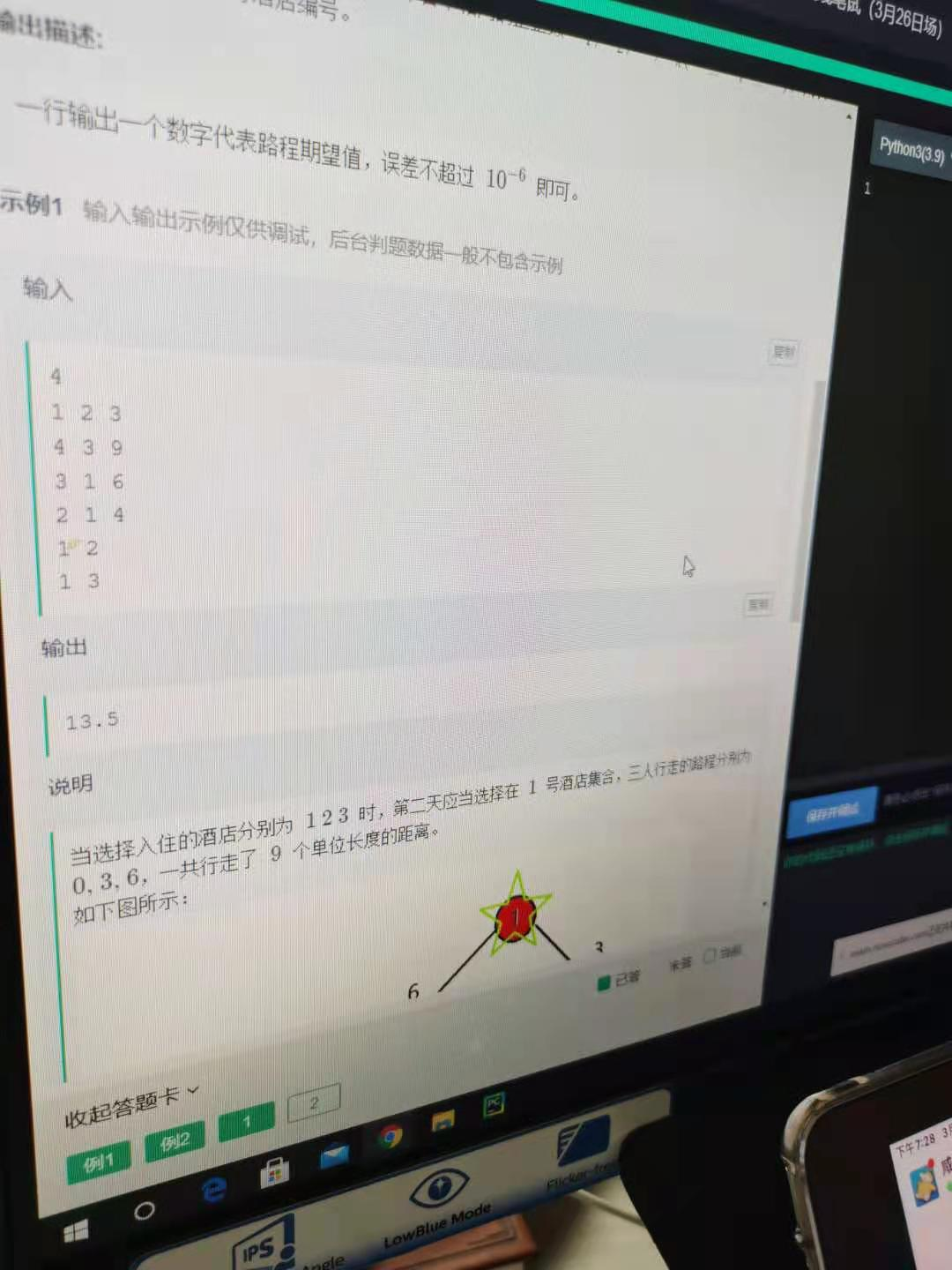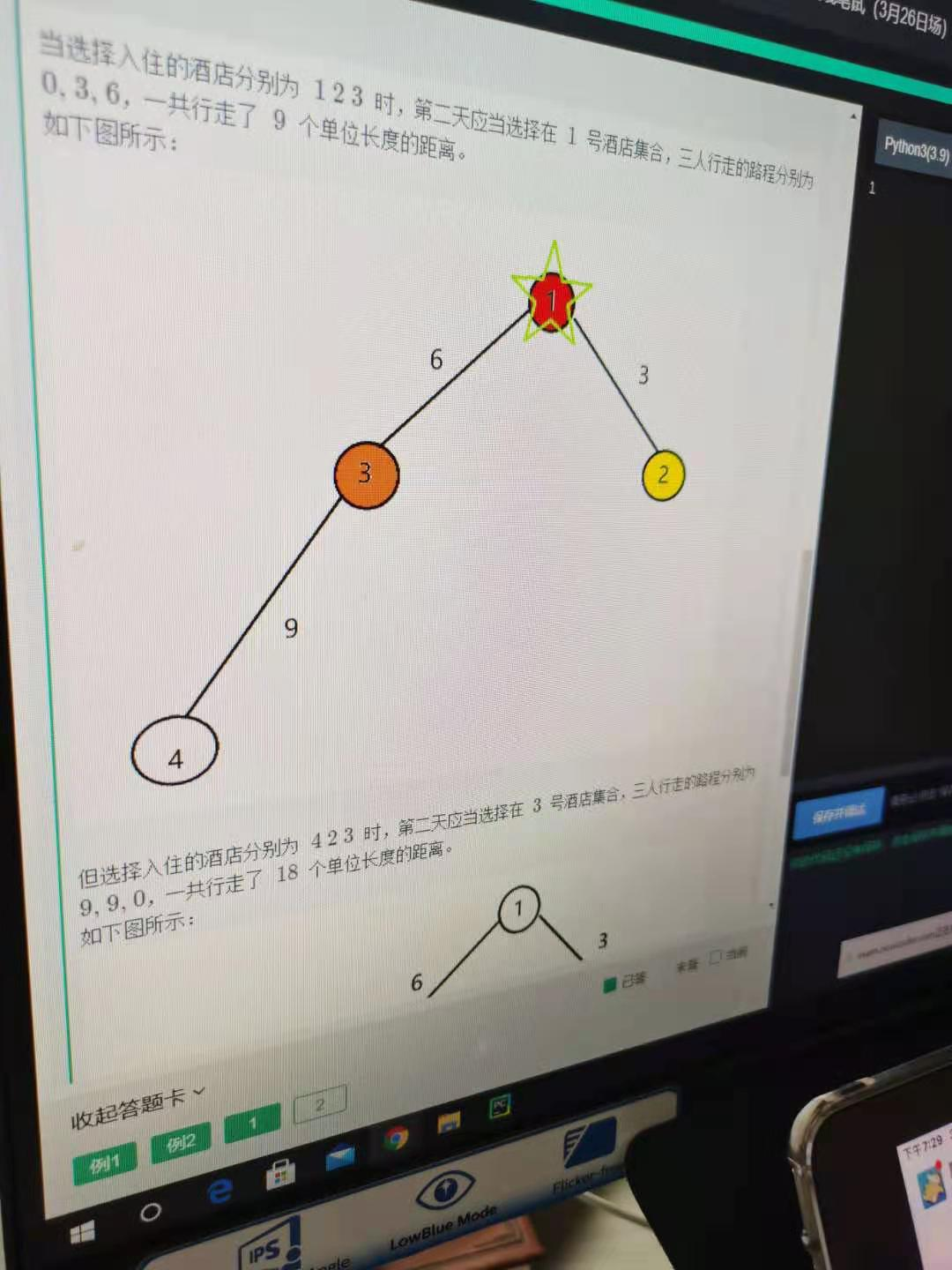1$x_i$表示一次实验边$i$产生的贡献，题目要求求$E[\sum_{i=1}^{n-1}x_i]=\sum_{i=1}^{n-1}E[x_i]$。接下来可以单独考虑每条边的贡献。

$A_i,B_i,C_i$表示顶点$i$子树下第$1,2,3$个人偏好的酒店数目。alihhh 求解答